# Sogang Computer Graphics Lab.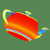On Using Curvature Characteristics for Polygonal Models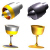Low Degree Approximation of Surfaces for Revolved Objects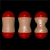Higher Order Interpolation and Least-Squares Approximation Using Implicit Algebraic Surfaces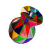Smoothing Polyhedra using Implicit Algebraic Splines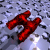Algebraic Surface Design with Hermite InterpolationPiecewise Linear Approximations of Digitized Space curves with Applications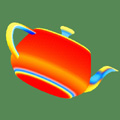On Using Curvature Characteristics for Polygonal Models
Abstract

This paper presents two measures that approximate surface curvature. The first measure, which can be proven to converge to the exact Gaussian curvature as we take finer polygonization, estimates the Gaussian curvature of various polygonal surfaces. This measure is geared up in an attempt to extract and visualize shape characteristics of polygonal surfaces and is shown to work effectively when exact mathematical equations of original surfaces are not known or are computationally expensive to handle. The second measure, although not so mathematically sound as the first one, is appropriate for an algorithmic process of adaptive polygonization. While it finds out easily how much a surface region bends, this measure is free of the intrinsic problems arisen in the Gaussian or mean curvatures. We adopt this measure in our new physically-based mesh generation algorithm that produces adaptively relaxed triangular meshes for parametric surfaces.

Paper
• I. Ihm, T. Park, Y. C. Wee, and S. Y. Shin, "On Using Curvature Characteristics for Polygonal Models", Computer Graphics International '94, pp.267-278, Melbourne, Australia, June 1994.
Image
1. Examples of the display of Gaussian curvature, approximated from the respective polygonized models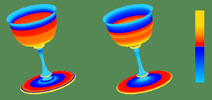and-continuous gobletsLow Degree Approximation of Surfaces for Revolved Objects
Abstract

We present a method for generating low degree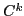-continuous piecewise algebraic surfaces for revolved objects. The approximating pieces are implicitly defined algebraic surfaces whose profile curves can be obtained algebraically or parametrically from digitized points. We show that degree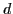surface patches can be used for approximations with inter-patchcontinuity as high as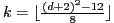for even, and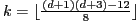for odd. As an example, we construct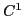cubic surfaces and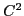quartic surfaces for revolved objects from digitized profile curves.

Paper
Image
1.cubic andquartic revolved surface models.
2.cubic andsextic revolved surface models.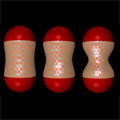Higher Order Interpolation and Least-Squares Approximation Using Implicit Algebraic Surfaces
Abstract

In this paper, we characterize the solution space of low degree, implicitly defined, algebraic surfaces which interpolate and/or least-squares approximate a collection of scattered point and curve data in three dimensional space. The problem of higher order interpolation and least-squares approximation with algebraic surfaces under a proper normalization reduces to a quadratic minimization problem with elegant and easily expressible solutions. We have implemented our algebraic surface fitting algorithms, and included them in the collaborative distributed geometric environment SHASTRA. Several examples are given to illustrate how our algorithms are applied to algebraic surface design.

Paper
• C. Bajaj, I. Ihm, and J. Warren, "Higher-Order Interpolation and Least-Squares Approximation Using Implicit Algebraic Surfaces," ACM Transactions on Graphics, Vol. 12, No. 4, pp. 327-347, October 1993.
Image
1. Acontinuous algebraic surface family of blobs.
2. Corner blending with algebraic surfaces.
3. SHILP: an algebraic surface editing tool.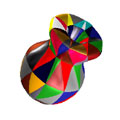Smoothing Polyhedra using Implicit Algebraic Splines
Abstract

Polyhedron "smoothing" is an efficient construction scheme for generating complex boundary models of solid physical objects. This paper presents efficient algorithms for generating families of curved solid objects with boundary topology related to an input polyhedron. Individual faces of a polyhedron are replaced by low degree implicit algebraic surface patches with local support. These quintic patches replace the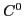contacts of planar facets withcontinuity along all interpatch boundaries. Selection of suitable instances of implicit surfaces as well as local control of the individual surface patches are achieved via simultaneous interpolation and weighted least-squares approximation.

Paper
Image
1. Smoothing a convex polyhedron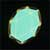a polyhedron with quartic wires: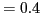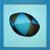aquintic algebraic patches: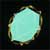a polyhedron with quartic wires: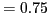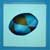aquintic algebraic patches:2. Smoothing a non-convex polyhedron with quintic algebraic patches
3. Two quintic algebraic patches with different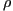values
4. A polyhedron smoothed in the SHASTRA Distributed and Collaborative Geometric Design Environment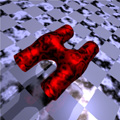Algebraic Surface Design with Hermite Interpolation
Abstract

This paper presents an efficient algorithm, called Hermite interpolation, for constructing low degree algebraic surfaces, which contain, withor tangent plane continuity, any given collection of points and algebraic space curves having derivative information. Positional as well as derivative constraints on an implicitly defined algebraic surface are translated into a homogeneous linear system, where the unknowns are the coefficients of the polynomial defining the algebraic surface. Computational details of the Hermite interpolation algorithm are presented along with several illustrative applications of the interpolation technique to construction of joining or blending surfaces for solid models as well as fleshing surfaces for curved wire frame models. A heuristic approach to interactive shape control of implicit algebraic surfaces is also given, and open problems in algebraic surface design are discussed.

Paper
• C. Bajaj, and I. Ihm, "Algebraic Surface Design with Hermite Interpolation", ACM Transactions on Graphics, Vol. 11, No. 1, pp. 61-91, January 1992.
• C. Bajaj, and I. Ihm, "Hermite Interpolation Using Real Algebraic Surfaces," The Fifth Annual ACM Symposium on Computational Geometry, 94-103, Saarbruchen, W. Germany, June 1989.
Image
1. Smooth joining of two cylinders with a cubic surface.
2. Smooth joining of three cylinders with a quartic surface.
3. "Good" and "bad" quartic joining surfaces.
4. Smooth joining of four cylinders with a quartic surface.
5. Smooth blending of two cylinders with a quartic surface.
6. Smooth blending of two cylinders with a quartic surface.
7. Smooth fleshing of a wire frame with quadric and quartic surfaces.
8. Interactive shape control using barycentric coordinates.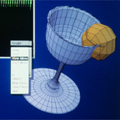Piecewise Linear Approximations of Digitized Space curves with Applications
Abstract

Generating piecewise linear approximations of digitized or densely-sampled curves is an important problem in many areas. Here, we consider how to approximate an arbitrary digitized 3-D space curve, made of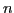points, with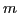line segments. We present an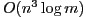time,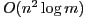space, dynamic programming algorithm which finds an optimal approximation. We then introduce an iterative heuristic algorithm, based upon the notions of curve length and spherical image, which quickly computes a good approximation of a space curve in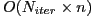time and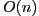space. We apply this fast heuristic algorithm to display space curve segments and implicit surface patches, and to linearly approximate curved 3D objects, made by rotational sweeping, by binary space partitioning trees that are well-balanced.

Paper
Image
1. Examples of the heuristic subdivision
2. Building a BSPT model of a goblet, partitioned through the heuristic subdivision method